## Flatten Binary Tree to Linked List

Given a binary tree, flatten it to a linked list in-place.
For example,
Given
         1
/ \
2   5
/ \   \
3   4   6

The flattened tree should look like:
   1
\
2
\
3
\
4
\
5
\
6

### Analysis:

Note that the problem requires in-place operation.The flatten procedure is like:  cut the left child and set to right, the right child is then linked to somewhere behind the left child. Where should it be then?  Actually the right child should be linked to the most-right node of the left node. So the algorithm is as follows:

(1) store the right child (we call R)

(2) find the right-most node of left child

(3) set R as the right-most node's right child.

(4) set left child as the right child

(5) set the left child NULL

(6) set current node to current node's right child.

(7) iterate these steps until all node are flattened.

### Code(Python):

/**
* Definition for binary tree
* struct TreeNode {
*     int val;
*     TreeNode *left;
*     TreeNode *right;
*     TreeNode(int x) : val(x), left(NULL), right(NULL) {}
* };
*/
class Solution {
public:
void flatten(TreeNode *root) {
while (root){
if (root->left){
TreeNode* pre=root->left;
while (pre->right){pre = pre->right;}
pre->right = root->right;
root->right = root->left;
root->left = NULL;
}
root=root->right;
}
}
};

### Code(Python):

# Definition for a  binary tree node
# class TreeNode:
#     def __init__(self, x):
#         self.val = x
#         self.left = None
#         self.right = None

class Solution:
# @param root, a tree node
# @return nothing, do it in place
def flatten(self, root):
while root != None:
if root.left == None:
root = root.right
else:
tmp = root.left
while tmp.right != None:
tmp = tmp.right
tmp.right = root.right
root.right = root.left
root.left = None



1.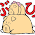I think the question requires an in-place algorithm.

Your answer does not seem to use only constant extra space.

1.Why doesn't it use constant extra space?

2.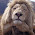Simple and elegant!

3.Nice solution!

4.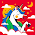What is the time complexity? Seems O(nlogn) to me.

5.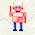Thanks for this neat solution. This is one simple optimization though. If the right subtree is null, there is no need to walk all the way down the left subtree to find the right most node and connect the right child of the right most node with the right subtree.

6.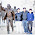This comment has been removed by the author.

7.Nice solution! What is the complexity?

8.So far, the best solution for this problems and also thanks for detailed analysis. Genius.

9.This comment has been removed by the author.

10.Your are visiting some of the nodes already visited
your complexity is > O(n)

This is my O(n) solution

/**
* Definition for a binary tree node.
* struct TreeNode {
* int val;
* TreeNode *left;
* TreeNode *right;
* TreeNode(int x) : val(x), left(NULL), right(NULL) {}
* };
*/
class Solution {
public:

void change(TreeNode* root, TreeNode** prev) {

if(!root) return;

cout << root->val<right = root;
(*prev)->left = NULL;
}

*prev = root;
TreeNode* left = root->left;
TreeNode* right = root->right;

change(left, prev);
change(right, prev);
}

void flatten(TreeNode* root) {

TreeNode* prev = NULL;
//*prev = NULL;

change(root, &prev);
}
};

1.Code is not clearly visible in above comment
11.